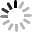## SNELL'S LAW OF REFRACTION

October 8, 2019

SNELL'S LAW OF REFRACTION

Before delving into each type of lens geometry, consider how optical lenses bend light using the property of refraction. Refraction is the means by which light is deviated by a certain amount when it enters or leaves a medium. This deviation is a function of the index of refraction of the medium and the angle the light makes with respect to the surface normal. This property is governed by Snell's Law of Refraction (Equation 1) where n1 is the index of the incident medium, θ1 is the angle of the incident ray, n2 is the index of the refracted medium, and θ2 is the angle of the refracted ray. Snell's Law describes the relationship between the angles of incidence and transmission when a ray travels between multiple media (Figure 2).

(1)n1sin(θ1)=n2sin(θ2)